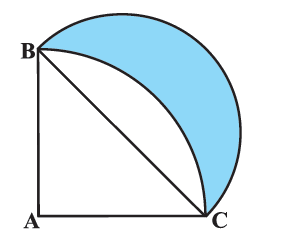# Ex.12.3 Q15 Areas Related to Circles Solution - NCERT Maths Class 10

Go back to  'Ex.12.3'

## Question

In Figure, $${ABC}$$ is a quadrant of a circle of radius $$14 \,\rm{cm}$$ and a semicircle is drawn with $${BC}$$ as diameter. Find the area of the shaded region.Video Solution
Areas Related To Circles
Ex 12.3 | Question 15

## Text Solution

What is known?

$$ABC$$ is a quadrant of a circle of radius $$\text{14 cm}$$ and a semicircle is drawn with $${BC}$$ as diameter.

What is unknown?

Reasoning:

To visualize the shaded region better mark point $$D$$ on arc $${BC}$$ of quadrant $${ABC}$$ and $$E$$ on semicircle drawn with $${BC}$$ as diameter.

From the figure it is clear that

Area of the shaded region $$=$$ Area of semicircle $${BEC}\, -$$ Area of segment $${BDC}$$

•  To find area of semicircle $${BEC},$$ we need to find radius or diameter$$(BC)$$ of the semicircle.

$$\Delta BAC$$ is a right angled $$\Delta$$, right angled at $${A}$$ ($${ABC}$$ being a quadrant)

Using Pythagoras theorem, we can find the hypotenuse ($${BC}$$)

• To find area of segment $$BDC$$

Area of segment $${BDC}\;=$$  Area of quadrant $${ABC} \,-$$ Area of $$\Delta \, {ABC}$$

Area of quadrant $${ABC}$$ can be found by using the formula

Area of sector of angle \begin{align}\theta = \frac{\theta }{{{{360}^\circ }}} \times \pi {r^2}\end{align}

$${\text{Area of }}\Delta ABC$$\begin{align}&= \frac{1}{2} \times {\rm{ base }} \times {\rm{ height }}\\&= \frac{1}{2} \times AC \times AB\left( {\angle A = {{90}^\circ }} \right)\end{align}

Steps:

$$\Delta {ABC}$$ is a right angled $$\Delta$$, right angled at $${A}$$

\begin{align} {BC^2} &= {AB^2} = {AC^2}\\ &= {14\rm{cm}^2} + {14\rm{cm}^2}\\ BC &= \sqrt {2 + {{14}^2}} \\ &= 14\sqrt 2 {\text{ cm}}\end{align}

$$\therefore\;$$Radius of semicircle $$BEC$$,\begin{align}r = \frac{BC}{2} = \frac{14\sqrt 2 }{2}\rm cm = 7\sqrt 2 cm\end{align}

Area of the shaded region= Area of semicircle $$BEC$$ - Area of segment $$BDC$$

Area of shaded region $$=$$  Area of semicircle $${BEC} \,–$$ (Area of quadrant  $${ABDC}\;-$$ Area $$\Delta {ABC}$$)

\begin{align}&= \frac{{\pi {r^2}}}{2} \!\!-\!\! \left( \frac{{90}}{{360}} \!\times\! \pi {{\left( {14} \right)}^2} \! -\! \frac{1}{2} \!\times\! AC \!\times\! AB \right) \\&= \frac{{\pi {{\left( \!{7\sqrt 2 } \right)}^2}}}{2} \!-\! \left(\frac{{{{\left( {14} \right)}^2}\pi }}{4} \!-\! \frac{1}{2}\! \times \!14 \!\times \!14\! \right)\\&= \frac{{22\! \times\! 7 \!\times\! 7 \times 2}}{{7 \times 2}}\!\! -\! \! \left( \! \frac{{22 \!\times\! 14 \!\times\! 14}}{{7 \!\times \!4}} \!-\! 7 \!\times \!14 \!\right) \\&= 154 - \left( {154 - 98} \right)\\&= 98 \rm{cm^2}\end{align}

Learn from the best math teachers and top your exams

• Live one on one classroom and doubt clearing
• Practice worksheets in and after class for conceptual clarity
• Personalized curriculum to keep up with school